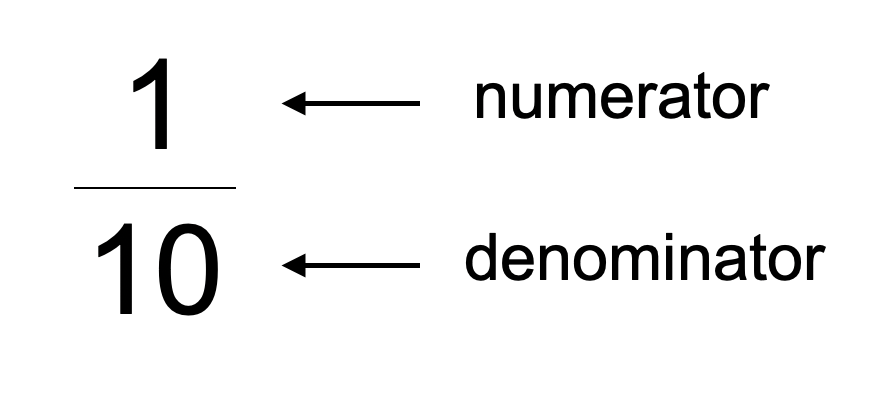# Counting in Tenths

## What is a tenth?

A tenth is one part of ten equal parts of a whole.This is how a tenth is written as a fraction:## Counting in tenths

When counting in tenths, the numerator increases by one each time, but the denominator stays the same.

## What comes after ten tenths?

Ten tenths is equivalent to one whole. Counting on from this, we have a whole number and a fraction, e.g. one and one tenth, one and two tenths, one and three tenths.

These can be recited and recorded as mixed fractions (see the top of the number line) or improper fractions (see the bottom of the number line):

## Counting in tenths Year 3

Counting in tenths appears in the National Curriculum in Year 3, in the Number - Fractions section:

• count up and down in tenths; recognise that tenths arise from dividing an object into 10 equal parts and in dividing one-digit numbers or quantities by 10

If you are looking for a ready-to-teach lesson that covers this objective, check out the first lesson in our Finding Fractions scheme, 'Counting in Tenths'.

## Tenths as decimals

This is how a tenth is written as a decimal fraction:

## Tenths as decimals Year 4

Tenths as decimals first appear in the statutory National Curriculum in Year 4, in the Number - Fractions (including decimals) section:

• recognise and write decimal equivalents of any number of tenths or hundreds

This objective is covered in our Fractions and Decimals lesson pack for year 4.